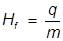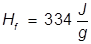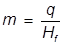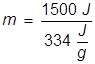# Heat of Fusion Formula

Heat of Fusion Formula

When a solid substance turns into a liquid substance it is known as melting. The melting process requires an increase in energy to allow the solid state particles to break free from each other. This input of energy is known as the heat of fusion. The heat of fusion is not the same for all substances, but is a constant value for each individual substance.Hf = heat of fusion

q = heat

m = mass

Heat of Fusion Formula Questions:

1. What is the heat of fusion for water if it takes 668 Joules of heat energy to melt 2.00 grams?2. What mass of water can be melted at 0°C if 1500 J of heat energy is applied?

The heat of fusion for water is applied here and the equation is rearranged to solve for mass.